Describing motion along a line

Start this free course now. Just create an account and sign in. Enrol and complete the course for a free statement of participation or digital badge if available.

Free course

# 6 Closing items

## 6.1 course summary

1. A coordinate system provides a systematic means of specifying the position of a particle. A system in one dimension involves choosing an origin and a positive direction in which values of the position coordinate increase. Values of the position coordinate are positive or negative numbers multiplied by an appropriate unit of length, usually the SI unit of length, the metre (m).

2. The movement of a particle along a line can be described graphically by plotting values of the particle's position x, against the corresponding times t, to produce a position-time graph. Alternatively, by choosing an appropriate reference position x﻿ref and defining the displacement from that point by s﻿x = xx ref, the motion may be described by means of a displacement-time graph.

3. Uniform motion along a line is characterised by a straight-line position-time graph that may be described by the equation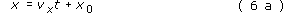where vx and x0 are constants. Physically, vx represents the particle's velocity, the rate of change of its position with respect to time, and is determined by the gradient of the position-time graph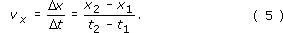x﻿0 represents the particle's initial position, its position at t = 0, and is determined by the intercept of the position-time graph, the value of x at which the plotted line crosses the x-axis, provided that axis has been drawn through t = 0.

4. Non-uniform motion along a line is characterised by a position-time graph that is not a straight line. In such circumstances the rate of change of position with respect to time may vary from moment to moment and defines the instantaneous velocity. Its value at any particular time is determined by the gradient of the tangent to the position-time graph at that time.

5. More generally, if the position of a particle varies with time in the way described by a function x﻿(﻿t﻿), then the way in which the (instantaneous) velocity varies with time will be described by the associated derived function or derivative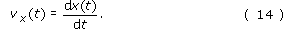6. The instantaneous acceleration is the rate of change of the instantaneous velocity with respect to time. Its value at any time is determined by the gradient of the tangent to the velocity-time graph at that time. More generally, the way in which the (instantaneous) acceleration varies with time will be described by the derivative of the function that describes the instantaneous velocity, or, equivalently, the second derivative of the function that describes the position: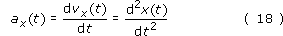7. Results and rules relating to differentiation and the determination of derivatives are contained in Table 6. The derivative of a constant is zero, the derivative of f﻿(﻿y﻿) = ﻿Ay﻿n﻿ is d﻿f﻿/﻿d﻿y = nAyn −1.

8. The signed area under a velocity-time graph, between specified values of time, represents the change in position of the particle during that interval.

9. Uniformly accelerated motion is a special case of non-uniform motion characterised by a constant value of the acceleration, ax = constant. In such circumstances the velocity is a linear function of time (v﻿x﻿(﻿t﻿) = u﻿x + a﻿x﻿t), and the position is a quadratic function of time (﻿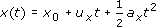).

10. The most widely used equations describing uniformly accelerated motion are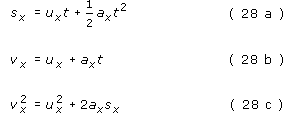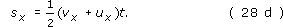11. Position x, displacement sx, velocity v x , and acceleration, ax, are all signed quantities that may be positive or negative, depending on the associated direction. The magnitude of each of these quantities is a positive quantity that is devoid of directional information. The magnitude of the displacement of one point from another, s = |﻿s﻿x﻿|, represents the distance between those two points, while the magnitude of a particle's velocity, v = |﻿v﻿x﻿|, represents the speed of the particle. The magnitude of the acceleration due to gravity is represented by the symbol g, and has the approximate value 9.81 m s﻿−﻿2 across much of the Earth's surface.

S207_2